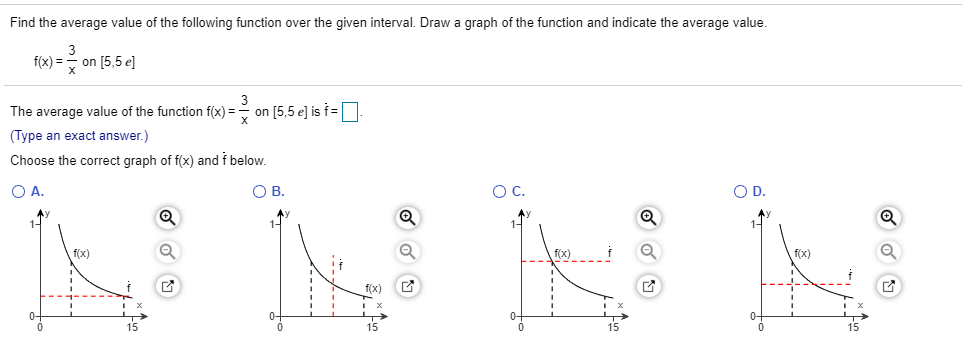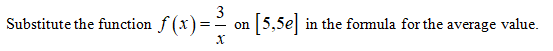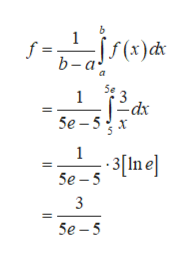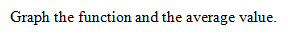# Find the average value of the following function over the given interval. Draw a graph of the function and indicate the average value.3fx)=on [5,5 elThe average value of the function f(x)=> on [5,5 e] is f=| |(Type an exact answer.)Choose the correct graph of f(x) and f belowO A.O C.O D.O Bf(x)fx)f(x)f(x)1515

Question
68 viewshelp_outlineImage TranscriptioncloseFind the average value of the following function over the given interval. Draw a graph of the function and indicate the average value. 3 fx)=on [5,5 el The average value of the function f(x)=> on [5,5 e] is f=| | (Type an exact answer.) Choose the correct graph of f(x) and f below O A. O C. O D. O B f(x) fx) f(x) f(x) 15 15 fullscreen
check_circle

Step 1help_outlineImage Transcriptionclosef = b-a(t)d 1 dx Se -5 х 1 Se In e 3 5e-5 fullscreen
Step 2...

### Want to see the full answer?

See Solution

#### Want to see this answer and more?

Solutions are written by subject experts who are available 24/7. Questions are typically answered within 1 hour.*

See Solution
*Response times may vary by subject and question.
Tagged in

### Integration